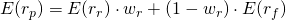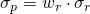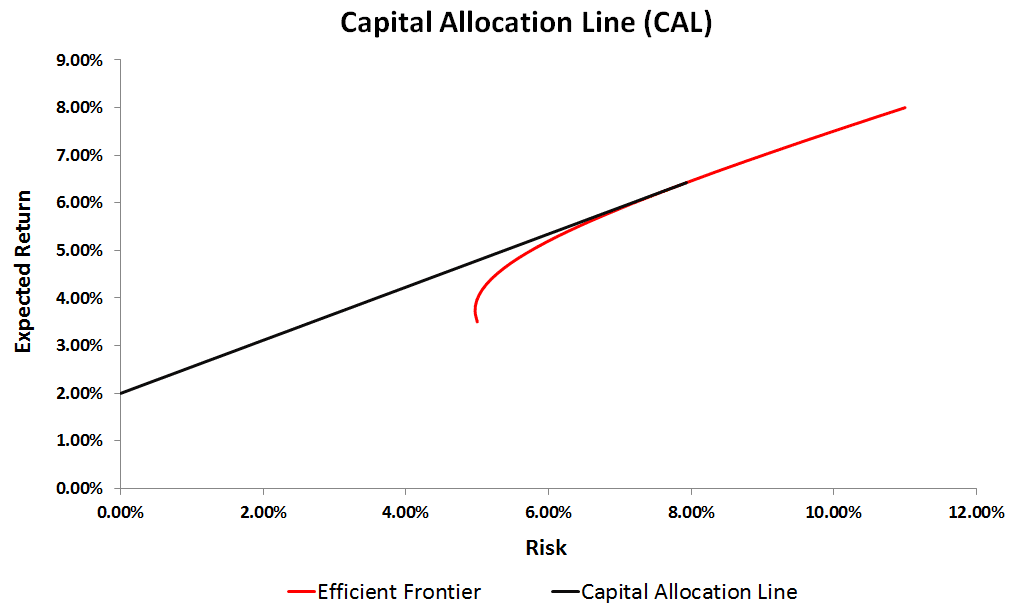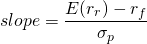# Capital Allocation Line (CAL)

The capital allocation line, which is sometimes also referred to as the Capital Market Line (CML), helps investors in deciding how much they ought to invest in the risky asset and the riskfree asset. The approach is fairly simple and can easily be implemented in Excel. At the bottom of this page, there’s an Excel file that constructs the Capital Market Line along with the efficient frontier. But first we explain the theory behind the CAL.

Let’s consider a very simple situation where we have two assets we can invest in. We can choose to invest in a risky portfolio (of shares) and a riskfree asset (such as a government bond). Now suppose both assets have the following characteristics.

Expected ReturnStandard Deviation
Risky Asset10%20%
Riskfree Asset5%0%

Since the riskfree asset is ‘riskless’, its standard deviation should be zero. Our portfolio of shares is risky, but it also yields a higher expected return to compensate investors for taking risk.

## Constructing portfolios

The basic question investors want to answer is how much to invest in both assets. To answer this question, lets first determine what the portfolio looks like if we combine both assets into a portfolio. Suppose the investor invests a fraction wr in the risky asset, and the rest (1-wr) in the riskless asset. In that case, the expected return of the portfolio (rp) can be determined using the following formulaThe riskiness of the portfolio rp can be calculated as followsThe formula for the riskiness of the portfolio is really simple because the standard deviation of the riskfree asset is zero.

Next, we make a figure of all the different combinations of both assets. The resulting line is called the capital allocation line (CAL). It shows all the possible asset allocations available to the investor.If we invest everything in the riskfree asset, our portfolio is riskless and we earn the return on the riskfree asset. As we move to the right, and invest more money in the risky asset, both the return and the riskiness of the portfolio go up. Finally, if we invest everything in the portfolio of stocks, we earn the expected return of the risky asset. In that case, the riskiness of the portfolio also equals the riskiness of the risky asset.

## Slope of the CAL

The slope of the capital allocation line equalsThis means that the slope measures the trade-off between return and risk. The steeper the capital allocation line, the higher the expected return investors receive in exchange for taking risk. This risk/return trade-off is referred to as the Sharpe ratio. Thus, the higher the Sharpe ratio of the risky portfolio, the more attractive the set of portfolios the investor can choose from. This means that we always prefer a steeper capital allocation line to a flatter one.

## Composition risky asset

Next, we need to determine the composition of the risky portfolio. From what we’ve learned above, we know it should have a Sharpe ratio that is as high as possible. That’s where Markowitz’s portfolio selection comes in.

## Summary

By combining a riskfree and a risky asset, we can construct portfolios that exhibit different levels of risk. That way, we can construct portfolios that meet investors’ needs. The Capital Market Line (CML) helps investors in allocating money between the risky- and the riskfree asset. The model can easily be implemented using Excel.